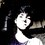# Math is getting broken.

the is some problem in log identities. please take a look : $f(x) = log \frac{x-i}{x+i}$ $g(x) = log \frac{x+i}{x-i}$ just by inspection we can conclude very easily that $f(x) = - g(x)$ but computing f(0) and g(0), we get $f(0) = log \frac{0-i}{0+i} = log(-1)$ $g(0) = log \frac{0+i}{0-i} = log(-1)$ for log(-1) we can use euler's identity that: $e^{i\theta} = cos\theta + isin\theta$ $for \theta = \pi$ $e^{i\pi} = -1$ taking log $ln(-1) = i\pi$ hence we got then : $f(0) = g(0) = i\pi$ this is contradicting f(x) = - g(x). i tried finding some limitations for loga - logb = log(a/b) identity on internet, but there are not.

so please tell me if there is some wrong in my computation or else.

i like complex numbers, and i think they are more than just imaginary.

by the way i got this problem while trying to compute the integral by partial fractions: $\int \frac{1}{x^{2}+1}dx = \frac{1}{2i} \int \frac{1}{x-i} - \frac{1}{x+i}dx$Note by Soham Zemse
6 years, 11 months ago

This discussion board is a place to discuss our Daily Challenges and the math and science related to those challenges. Explanations are more than just a solution — they should explain the steps and thinking strategies that you used to obtain the solution. Comments should further the discussion of math and science.

When posting on Brilliant:

• Use the emojis to react to an explanation, whether you're congratulating a job well done , or just really confused .
• Ask specific questions about the challenge or the steps in somebody's explanation. Well-posed questions can add a lot to the discussion, but posting "I don't understand!" doesn't help anyone.
• Try to contribute something new to the discussion, whether it is an extension, generalization or other idea related to the challenge.
• Stay on topic — we're all here to learn more about math and science, not to hear about your favorite get-rich-quick scheme or current world events.

MarkdownAppears as
*italics* or _italics_ italics
**bold** or __bold__ bold
- bulleted- list
• bulleted
• list
1. numbered2. list
1. numbered
2. list
Note: you must add a full line of space before and after lists for them to show up correctly
paragraph 1paragraph 2

paragraph 1

paragraph 2

[example link](https://brilliant.org)example link
> This is a quote
This is a quote
    # I indented these lines
# 4 spaces, and now they show
# up as a code block.

print "hello world"
# I indented these lines
# 4 spaces, and now they show
# up as a code block.

print "hello world"
MathAppears as
Remember to wrap math in $$ ... $$ or $ ... $ to ensure proper formatting.
2 \times 3 $2 \times 3$
2^{34} $2^{34}$
a_{i-1} $a_{i-1}$
\frac{2}{3} $\frac{2}{3}$
\sqrt{2} $\sqrt{2}$
\sum_{i=1}^3 $\sum_{i=1}^3$
\sin \theta $\sin \theta$
\boxed{123} $\boxed{123}$

## Comments

Sort by:

Top Newest

Log doesn't apply to negative number.

What is log 0 ? ( let base be 10)

Log 0 = x => 0 = 10^x

X has to be -infinity for this to hold true.

So 0 is limit. You can't go below 0. Technically speaking log 0 is also undefined. And so is log (-1)

So our general rule won't hold true.

Limitation : log applies only to positive real numbers

- 6 years, 11 months ago

Log in to reply

hi,

yes in real calculus, log of negative number is not defined, but this doesn't stops us from seeking further,

you can have a read on http://en.wikipedia.org/wiki/Complex_logarithm

in fact when a number is not a positive real number, its logarithm is a complex number. eg : $z=r e^{i\theta}$ then if we could take natural log (base e) on both side, $ln z = ln r + i\theta$ where r is the modulus of z and theta is principal argument.

- 6 years, 11 months ago

Log in to reply

\sqrt { a*b } =\sqrt { a } *\sqrt { b } holds if and only if any one of a and b is non negative.... thus your second step where you cancel -i/i to get -1 is wrong because both numerator and denominator have negative real numbers hence the rule stated earlier cannot be used...... hence value of f(0)= log(1) and not log(-1).

- 6 years, 11 months ago

Log in to reply

i just multiplied and divided by i in (-i/i) to get (1/-1) = -1. anyways thanks for that rule, it was troubling me.

- 6 years, 11 months ago

Log in to reply

try this now! {its an interesting paradox like the one you shared above}.. here's the link:

{ https://brilliant.org/discussions/thread/beware-of-blind-algebra/} .......... i would also request you to reshare this as i have a poor following :p........... thanks!!

- 6 years, 11 months ago

Log in to reply

×

Problem Loading...

Note Loading...

Set Loading...